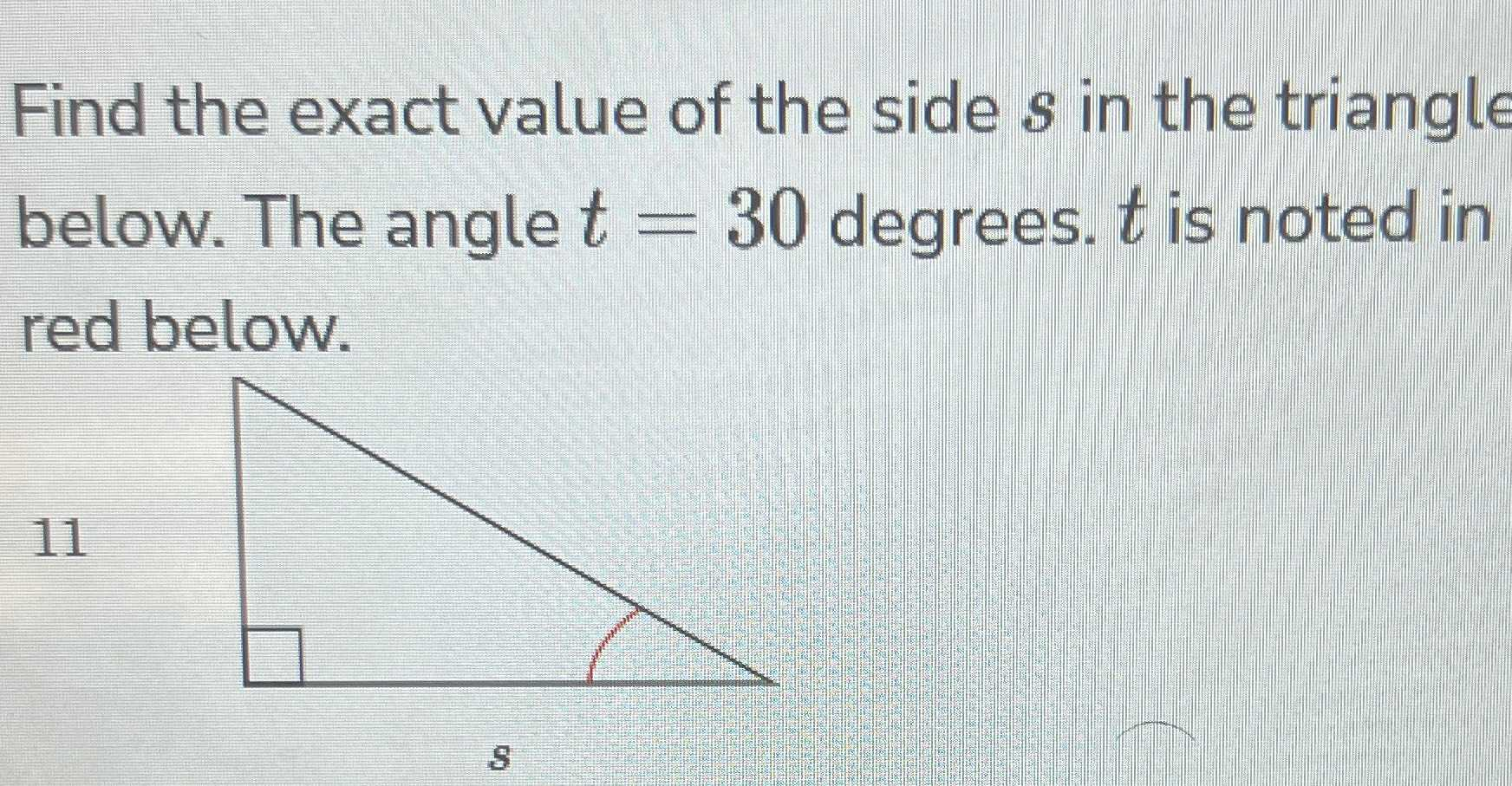### Still have math questions?

Trigonometry
QuestionFind the exact value of the side $$s$$ in the triangle below. The angle $$t = 30$$ degrees. $$t$$ is noted in red below.

$$tan30° = \frac{11}{s}$$